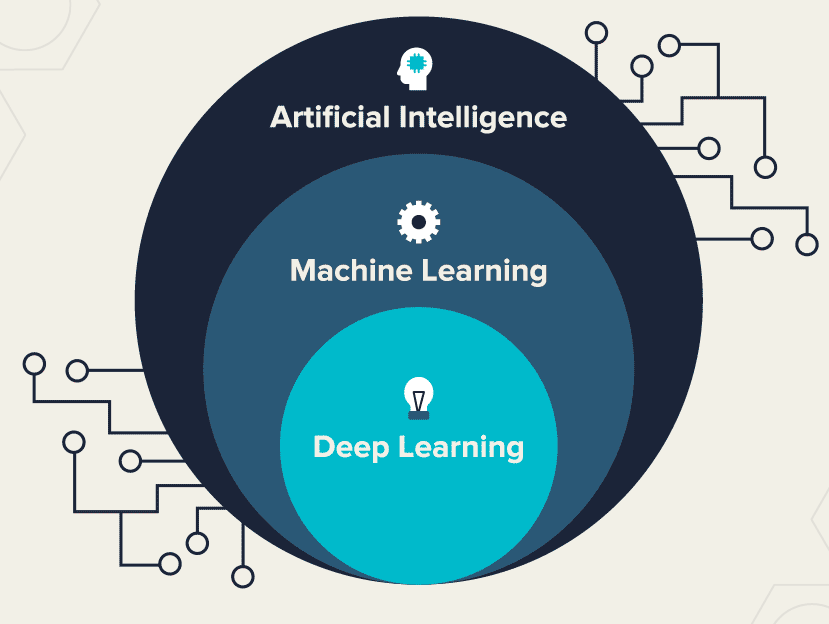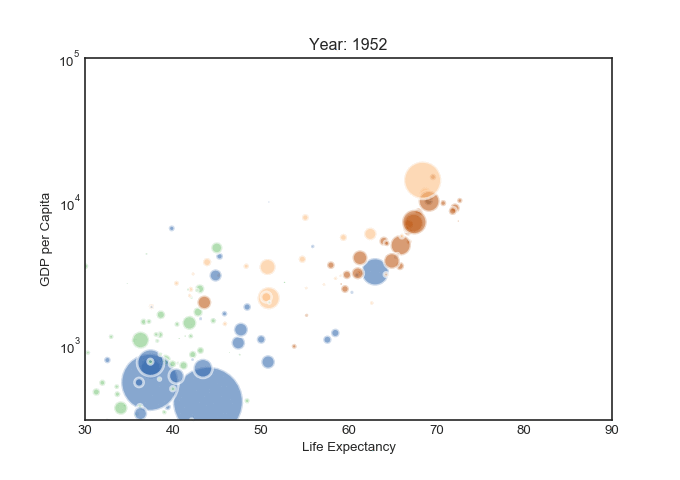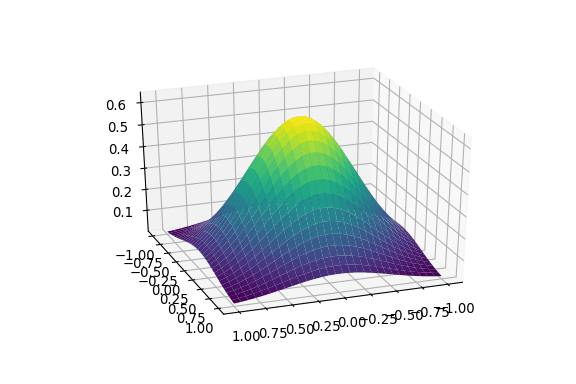# Beginners’ cookbook for Machine Learning recipes

A comprehensive theoretical+practical introduction to Machine Learning for newbies

In the world of technology, the terms “Machine Learning”, “Deep Learning”, and “Artificial Intelligence” have become quite the buzzwords, and rightly so because just as the internet was a revolution a couple of decades ago, the same is the case here. The world’s technology is expected to be fully adapted to the automated regime in the next few years, so why would you want to miss out on all the hype?

This article is an attempt to introduce the concept of machine learning to beginners who have more or less no idea about the practicality of it but are interested in knowing where to get started, so let’s do it!

# What is Machine Learning (ML)?

To put it very simply, Machine Learning is a subset of Artificial intelligence in which we “train” the computer to decide rules for solving a particular problem at hand, based on the given data.

For example, when children enter kindergarten, they are taught how to read alphabets and numbers, and with time they learn to distinguish between them based on their unique shapes. That is exactly what the thought process behind ML is! We are supposed to make the computer learn as if it was similar to a human brain by exposing it to real-life examples.Deep Learning is a subset of Machine Learning, which is a subset of Artificial Intelligence

# Problems to be solved

While there are many different sub-types of problems that can be solved by implementing machine learning algorithms, for a beginner, it is sufficient to broadly define them into two categories:

1. Regression: Problems where we need a prediction to be made on a continuous scale, in other words, prediction of a real value, for example, forecasting the price of the stock market for a particular company for the next 30 days, or temperature in the upcoming week, or the housing prices of a particular city on the basis of area, number of floors, date of construction, etc.

2. Classification: Problems where we need to define our output as part of a category or a class, for example, species of flowers based on petal length, sepal width, stem height, etc. or differentiating between a cat and a dog, or even a cat and a human.

At this point, it is important to mention that clustering is another type of problem that we encounter more often than not which is similar to classification problems but the difference between the two is that clustering does not use predefined classes (target values) in order to make its predictions. It groups data based on similarities between the values of the features.

# Learning Algorithms

Now that we have discussed the type of problems we might come across, we also need to know how we can solve them. For this, I would like to introduce you to the various learning algorithms, which are as follows:

1. Supervised Learning: When going through data, we usually see a list of features that we use to make our predictions. In supervised learning, these target values are also part of the data, with the help of which we train the machine to select an appropriate value for our predictions. For example, in a classification problem in which we need to recognize the spoken words from an audio file, the value of said words will be there to guide us in the right direction.
2. Unsupervised Learning: Sometimes, we do not have target values with us, in which case we use the machine to analyze and group the data itself while forming a tangible pattern. For example, if we have to predict whether a news article is fake or not, the machine can use the concepts of clustering to find noticeable features which help differentiate between the two categories without explicitly telling the machine what we want, hence the name “unsupervised”.
3. Reinforcement Learning: Problems in which a computer is needed to fulfill a requirement, or meet a certain goal by forming strategies or making decisions as if it was in a game-like situation, which can be simple or a complex environment. This is done with the help of “rewards” and “punishments” assigned after every repetition of the algorithm. For example, calculating the trajectory of an object, or automating the process of parking a car can be achieved with the help of reinforcement learning.

These are only the algorithms that we use to solve our problems. Within these algorithms, we have many models that we can implement based on our needs.

# Visualization

It is a well-known fact that humans learn better and faster if they are able to visualize things. So before we “crunch the numbers” it is always advisable, especially if you are a beginner, to visualize the data and get as many inferences from it as you can. There are numerous ways of depicting data using various libraries such as seaborn and matplotlib in python. Below are two such examples:2-dimensional data representing life expectancy in relation to GDP per capita with each frame representing a yearA 3-dimensional plot which is showing a contour. Sometimes 2-D plots are not able to do justice to the type of data that we might have.

# Preprocessing

Perhaps the most important part of machine learning. It can be compared to the human body in the sense that if we want our body to run efficiently, or give a proper output, so to speak, we need our food to be properly cooked.

Similarly, before giving some data to our model, we need to make sure that data is in the proper form so that the model can interpret and run calculations on it while giving the most desirable results. The following techniques are usually used to preprocess the data:

1. Data cleaning: Often, real-world data is considered to be full of “noise” which needs to be “cleaned”. This can include missing data values that need to be filled, outliers that may negatively affect the results, inconsistency in data entry formatting, which could be a human or computer error, etc.

Some methods of dealing with these issues include:

b)fill null values using aggregation techniques

c) reviewing outlier values by plotting a boxplot and removing them

d) formatting errors can be reviewed manually and rectified

2. Data Integration: Let’s say that data from one source is not enough to train your model, so we decide to use multiple sources. Integrating data from all these sources means reviewing redundant and irrelevant values. It can be a little tricky as data formats might be dissimilar and each of them might require different data cleaning techniques.

3. Data transformation: This part of preprocessing deals with changing the values of features in a way that they are comparable to each other. Features in data can be in two popular forms: numerical(continuous values) and categorical (data in the form of classes). Dealing with such data can be done in many ways, the two most common ones are:

a) Normalization/Standardization: When the range of data is quite large, or uneven between features, it is always advisable to compress it in a common range for the sake of uniformity.

b) Label/One-hot Encoding: When the available features take the form of classes or categories, we encode them in a format that is readable by the machine, which is, of course, numbers. The table below explains the difference between Label encoding and One-hot encoding. In the former, different values are assigned to different categories, whereas in the latter, a different feature column is assigned in a binary vector-like manner to each category.

4. Data reduction/Feature extraction: Sometimes, not all features are relevant, which can cause problems especially when the dataset is large in size. This can lead to the process becoming slower, or our model becoming negatively influenced and providing inaccurate results. For example, while predicting the potential marks of students in their final year based on their previous years’ scores, we do not need to know their enrollment number, so we choose to remove that column. There are various techniques to help achieve this, such as:

a) Forward selection: We start with having no feature in the dataset, then we add them one by one while analyzing the performance of the model. We keep going as long as the performance improves, and we stop if it does not change or becomes worse.

b) Backward elimination: This can be considered as an opposite approach to forward selection, where we start out with the complete dataset, then we remove one feature at a time till we see an improvement in performance.

c) Recursive feature elimination: This is a greedy technique which undergoes several iterations and stores the best and worst features in each iteration and improves the model accordingly. It ranks the features in order of their relevance.

# Models

The word “model” has been used quite a few times in the previous sections, so now let us learn what it actually means. The model is that portion of our machine learning program which “crunches the numbers” so to speak. It works on the training data and assigns “weights” to the features which help in making efficient predictions. There are a wide array of models that one can choose from, depending on the project, and there is an immense amount of mathematical understanding needed if you want to understand what the model really does. But the aim of this article is to be beginner-friendly so keeping that in mind, I am just going to list out a few models (with their python documentation link) that can help you get started on your data science adventures!

1. Regression:

2. Classification:

# Metrics

The job is not finished after training the model, in fact, this is the part where humans have a more important role to play than computers i.e. the analysis of results. Various metrics are used to measure the efficiency of the model, depending on our problem statement. Some of the most popular analysis methods are as follows:

1. Confusion Matrix: This simple-to-interpret matrix helps in analysing the correctness of our classifications by printing an array like the one given below.Confusion matrix is given in the form of a “heatmap”. The darker the cells are in the diagonal elements (or the greater the numbers), the more accurate are the predicted values

2. Accuracy: It is the ratio of number of correctly predicted elements and total number of elements, which can be written as

Accuracy= (True Positive+True Negative)/(True Positive +False Positive+True Negative+False Negative)

3. Precision: Accuracy can often be misleading, especially when the dataset does not have a balanced amount of values that need to be predicted, for this we adopt methods like precision, which means:

Precision= (Number of True Positive values)/(True Positive+ False Positive values)

4. Recall: This is the fraction of samples from the same class that is correctly predicted, given by:

Recall= (True Positive)/ (True Positive+ False Negative)

5. F1-score: This metric combines precision and recall to give a balanced output of our efficiency.

6. Mean Squared Error: Perhaps the most commonly used regression metric is MSE, which is used to show the deviation of our model’s predicted value from the ground truth.

7. Mean Absolute Error: This metric calculates the absolute difference between the predicted and actual values. While MSE gives a clearer understanding of how our predicted values compare to the actual ones, MAE is more robust in the sense that it can handle the problem of outliers, unlike MSE which tends to give higher weightage to them, thus negatively affecting our model.

Documentation for python codes of various metrics can be found here.

# Conclusion

Phew! That was quite an introduction, but if you read thoroughly, with a basic understanding of python or any other language of your preference, you can start experimenting, after all, experience is the best teacher in this field.

Feel free to comment below in case you have any doubts!